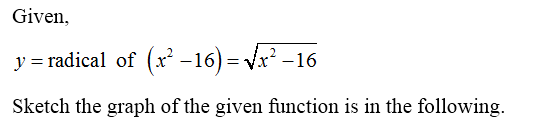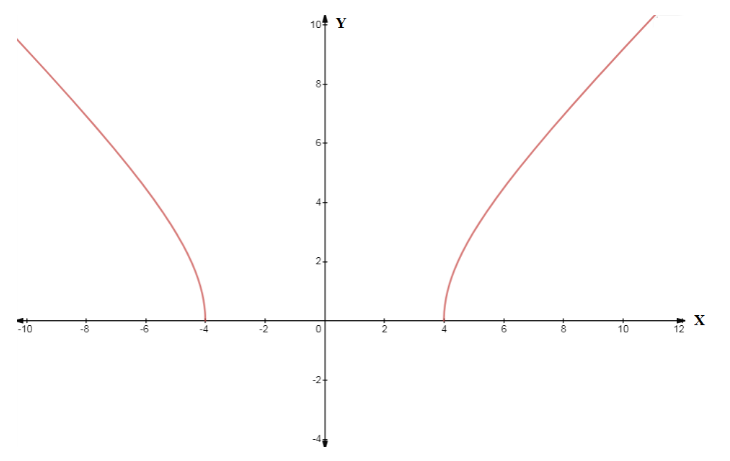# Find the x- and y-intercepts of the graph of the equation. (If an answer does not exist, enter DNE.)(a)    y = radical of x2 − 16(sorry i dont know how to type the radical in here? i made the first two, but i did not made the third one, please help)x-intercept    (x, y) =     -4, 0     (smaller x-value)x-intercept    (x, y) =     4,0      (larger x-value)y-intercept    (x, y) =

Question
1 views

Find the x- and y-intercepts of the graph of the equation. (If an answer does not exist, enter DNE.)

(a)
 x2 − 16
x-intercept     (x, y)  =

-4, 0
(smaller x-value)
x-intercept     (x, y)  =

4,0

(larger x-value)
y-intercept     (x, y)  =

check_circle

Step 1Step 2...

### Want to see the full answer?

See Solution

#### Want to see this answer and more?

Solutions are written by subject experts who are available 24/7. Questions are typically answered within 1 hour.*

See Solution
*Response times may vary by subject and question.
Tagged in